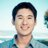# Calling R from Scala sbt projects

[Update: The jvmr package has been replaced by the rscala package. There is a new version of this post which replaces this one.]

### Overview

In previous posts I’ve shown how the jvmr CRAN R package can be used to call Scala sbt projects from R and inline Scala Breeze code in R. In this post I will show how to call to R from a Scala sbt project. This requires that R and the jvmr CRAN R package are installed on your system, as described in the previous posts. Since I’m focusing here on Scala sbt projects, I’m also assuming that sbt is installed.

The only “trick” required for calling back to R from Scala is telling sbt where the jvmr jar file is located. You can find the location from the R console as illustrated by the following session:

```&gt; library(jvmr)
&gt; .jvmr.jar
 "/home/ndjw1/R/x86_64-pc-linux-gnu-library/3.1/jvmr/java/jvmr_2.11-2.11.2.1.jar"
```

This location (which will obviously be different for you) can then be added in to your sbt classpath by adding the following line to your build.sbt file:

```unmanagedJars in Compile += file("/home/ndjw1/R/x86_64-pc-linux-gnu-library/3.1/jvmr/java/jvmr_2.11-2.11.2.1.jar")
```

Once this is done, calling out to R from your Scala sbt project can be carried out as described in the jvmr documentation. For completeness, a working example is given below.

### Example

In this example I will use Scala to simulate some data consistent with a Poisson regression model, and then push the data to R to fit it using the R function glm(), and then pull back the fitted regression coefficients into Scala. This is obviously a very artificial example, but the point is to show how it is possible to call back to R for some statistical procedure that may be “missing” from Scala.

The dependencies for this project are described in the file build.sbt

```name := "jvmr test"

version := "0.1"

scalacOptions ++= Seq("-unchecked", "-deprecation", "-feature")

libraryDependencies  ++= Seq(
"org.scalanlp" %% "breeze" % "0.10",
"org.scalanlp" %% "breeze-natives" % "0.10"
)

resolvers ++= Seq(
"Sonatype Snapshots" at "https://oss.sonatype.org/content/repositories/snapshots/",
"Sonatype Releases" at "https://oss.sonatype.org/content/repositories/releases/"
)

unmanagedJars in Compile += file("/home/ndjw1/R/x86_64-pc-linux-gnu-library/3.1/jvmr/java/jvmr_2.11-2.11.2.1.jar")

scalaVersion := "2.11.2"
```

The complete Scala program is contained in the file PoisReg.scala

```import org.ddahl.jvmr.RInScala
import breeze.stats.distributions._
import breeze.linalg._

object ScalaToRTest {

def main(args: Array[String]) = {

// first simulate some data consistent with a Poisson regression model
val x = Uniform(50,60).sample(1000)
val eta = x map { xi =&gt; (xi * 0.1) - 3 }
val mu = eta map { math.exp(_) }
val y = mu map { Poisson(_).draw }

// call to R to fit the Poission regression model
val R = RInScala() // initialise an R interpreter
R.x=x.toArray // send x to R
R.y=y.toArray // send y to R
R.eval("mod &lt;- glm(y~x,family=poisson())") // fit the model in R
// pull the fitted coefficents back into scala
val beta = DenseVector[Double](R.toVector[Double]("mod\$coefficients"))

// print the fitted coefficents
println(beta)

}

}
```

If these two files are put in an empty directory, the code can be compiled and run by typing sbt run from the command prompt in the relevant directory. The commented code should be self-explanatory, but see the jvmr documentation for further details.### darrenjw

I am Professor of Stochastic Modelling within the School of Mathematics & Statistics at Newcastle University, UK. I am also a computational systems biologist.

## 6 thoughts on “Calling R from Scala sbt projects”

1.Kevin Tham (@kevinsf90) says:

Have you tried whether you can use ggplot2 from Scala?

2.shouhengyi says:

You might find this very interesting. It’s a presentation of scaling R by using Scala’s Akka framework 🙂

3.saskoder says:

Found this site few days back and am really blessed! I was thinking for sometime about R and Scala integration to bypass python. Thanks Sir for the enlightening post!

4.Georg Heiler says:

Can you compile the program using scala 2.10?
because for me it results in the following problem:

[error] found : R.type (with underlying type org.ddahl.rscala.callback.RClien
t)
[error] required: ?{def x: ?}
[error] Note that implicit conversions are not applicable because they are ambig
uous:
[error] both method any2Ensuring in object Predef of type [A](x: A)Ensuring[A]
[error] and method any2ArrowAssoc in object Predef of type [A](x: A)ArrowAssoc[
A]
[error] are possible conversion functions from R.type to ?{def x: ?}
[error] R.x = x.toArray // send x to R
[error] ^
[error] one error found
[error] (compile:compileIncremental) Compilation failed
[error] Total time: 25 s, completed 01.05.2016 19:44:09
A minimal example can be found here https://github.com/scalaj/scalaj-http but the same problem remains for any R code I try to execute for scala 2.10

This site uses Akismet to reduce spam. Learn how your comment data is processed.